Home

 << Previous    1...   11  12    14  15  ...24    Next >>

13. Square representations of some parameters of the 421 polytope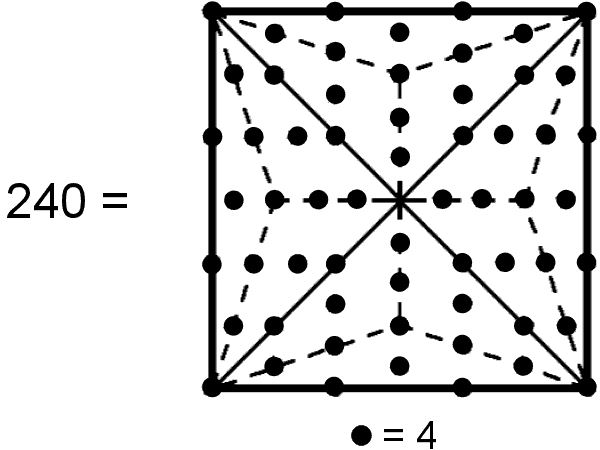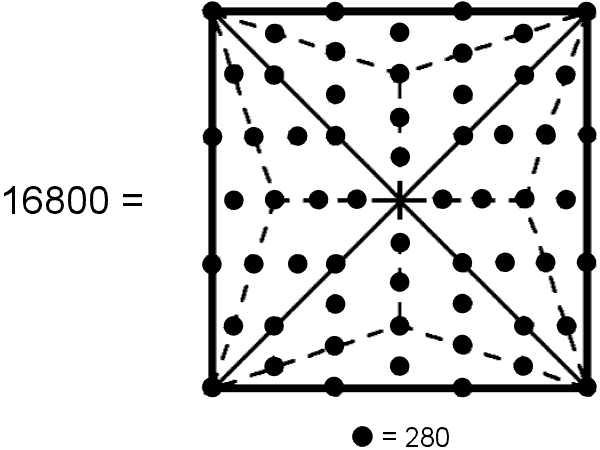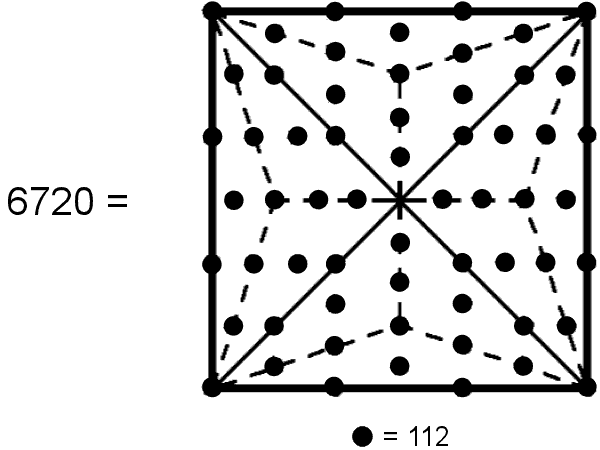Weighted with the Tetrad (4), the 60 yods surrounding the centre of the Type B square generate the number of vertices in the 421 polytope. A Weighted with the number value 280 of Sandalphon, the Archangel of Malkuth, the 60 yods surrounded the centre of the Type B square generate the superstring structural parameter 16800. B Weighted with the number value 112 of BeniElohim, the Order of Angels assigned to Hod, the 60 yods surrounding the centre of the Type B square generate the number (6720) of edges in the 421 polytope. C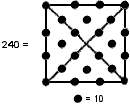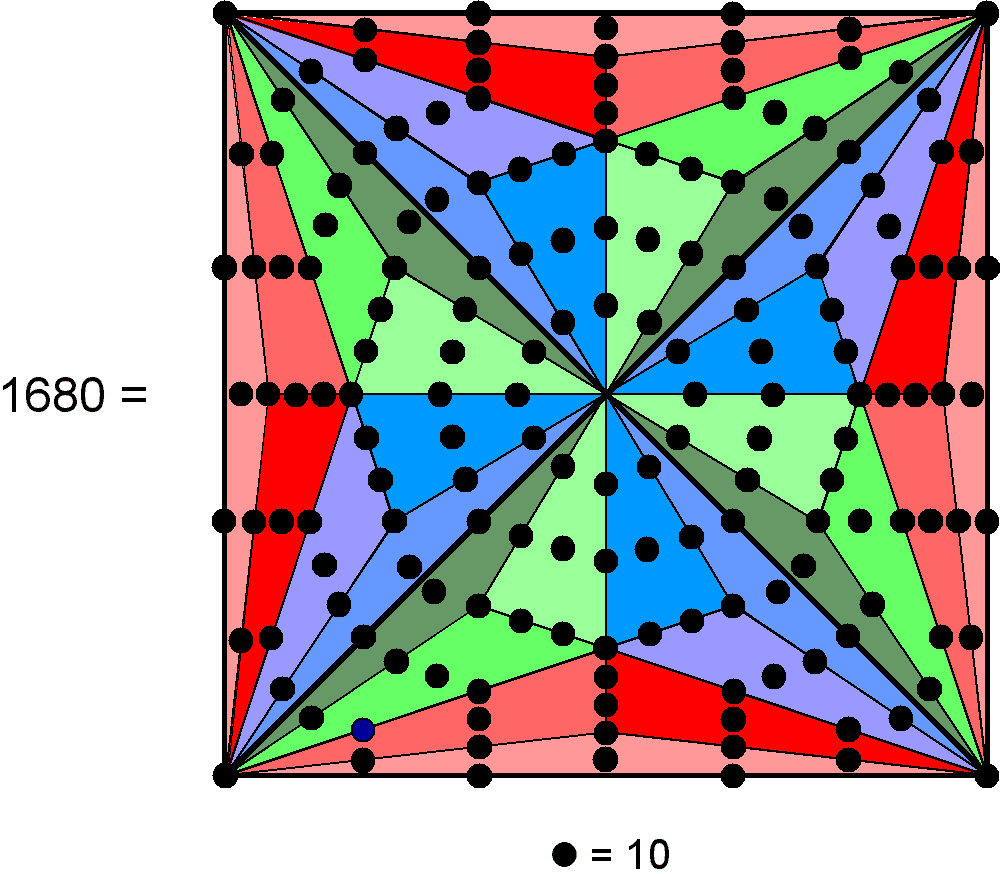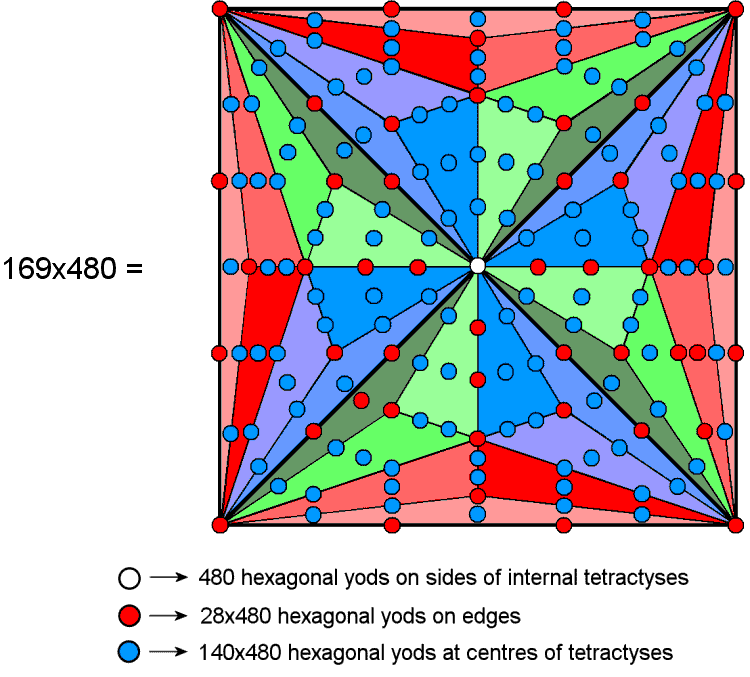Weighted with the Decad (10), the 24 yods surrounding the centre of the Type A square generate the number (240) of vertices in the 421 polytope. Weighted with the Decad, the 168 yods surrounding the centre of the Type C square generate the superstring structural parameter 1680. Weighted with the number (480) of roots of E8×E8, the 169 yods in the Type C square generate the number (169×480) of hexagonal yods in the 421 polytope. D E F

×Figure A
Sixty yods surround the centre of the Type B square. Weighted with the Tetrad, whose geometrical symbol is the square, they generate the number 240. This is the number of vertices in the 421 polytope. As the geometrical symbol of the Tetrad (4), the square implements par excellence the Tetrad Principle governing parameters of holistic systems.

Figure B
Weighted with the number value 280 of Sandalphon, the Archangel of Malkuth, the Type B square generates the number 16800. This is the number of turns in the 10 helical whorls of the UPA, the subquark state of the E8×E8 heterotic superstring.

Figure C
The Type B square generates the number of edges of the 421 polytope when the number value 112 of Beni Elohim, the Order of Angels assigned to Hod, is the weight of the 60 yods surrounding its centre.

Figure D
Twenty-four yods (=4!) surround the centre of the Type A square. With each yod weighted with the Decad (10) as the sum of the first four integers, the 24 yods generate the number 240. This is the number of vertices of the 421 polytope.

Figure E
The Type C square has 169 yods. Weighted with the Decad, the 168 yods surrounding its centre generate the number 1680 as the number of turns in each helical whorl of the UPA/subquark state of the E8×E8 heterotic superstring.

Figure F
As we found earlier, (8×1680=28×480) hexagonal yods line the 6720 edges of the 421 polytope. (36×1680=126×480) hexagonal yods are at the centres of its faces and (4×1680=14×480) hexagonal yods are at the centres of its internal tetractyses. Hence, (126+14=140)×480 hexagonal yods are at centres of tetractyses, where 140 is the number value of Melekim, the Order of Angels assigned to Tiphareth. The former can be represented by the 28 yods either lining the sides of the Type C square or at corners of tetractyses; the latter can be represented by the remaining 140 hexagonal yods.

 << Previous    1...   11  12    14  15  ...24    Next >>

Home